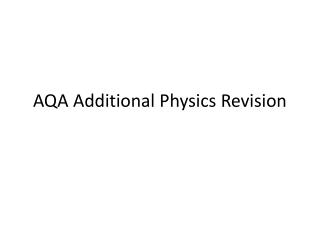# AQA Additional Physics Revision - PowerPoint PPT PresentationDownload PresentationDownload Presentation- - - - - - - - - - - - - - - - - - - - - - - - - - - E N D - - - - - - - - - - - - - - - - - - - - - - - - - - -
##### Presentation Transcript

2. Know how to:Read distance - time graphs

3. Read velocity – time graphs

4. How do you calculate acceleration from a speed-time graph?Acceleration = change in velocity/time taken for the changee.g. 10m/s = 2m/s2 5s

5. Be able to plotVelocity-time graphs • Bbcbitesize • Science > Additional Science (AQA) > Forces and motion > Representing motion

6. Understand terminal velocity

7. Weight= Mass x gravitational field strength

8. The factors affecting the stopping distance of a vehicle

9. Work = force x distance moved in direction of force

10. F=MA • See bbcbitesize additional science aqa physics • Forces and motion

11. Elastic potential energy is stored in an object when a force change its shape

12. Kinetic energy depends on speed and mass

13. Kinetic energy= ½ x mass x speed • Bbcbitesize • Science > Additional Science (AQA) > Forces and motion > Kinetic energy

14. When a force acts on a body a change in momentum occursMomentum is conserved in any collision/explosion provided no external forces act

15. To use the idea of momentum to explain safety features

16. Force = change in momentum/ time taken

17. How materials become charged by friction

18. Electrically charged bodies exert forces on each other;LIKE CHARGES REPELUNLIKE CHARGES ATTRACT

19. Van de Graaff generator

20. Simple diagrams showing how a lightning conductor works Lightning conductors work to protect tall buildings from lightning strike. This is by providing an easier path for current to flow to the earth other than through the building. It consists of a thick copper strip of very low resistance connected to a large metal plate deep in the damp earth.

21. How a photocopier works

22. How a smoke precipitator works

23. Meaning of series and parallel

24. graphs for a resistor at constant temperature, a filament lamp and a Diode

25. Resistance graphs

26. Potential difference (V)=current(A) X resistance(Ohms)V=IR • Pd Current resistance • 2v .......... 2 ohms • ......... 1.5 amps 2 ohms • 4v 2 amps .......... • .......... 3 amps 2 ohms • A p.d. of 4v is applied to two resistors (6 ohms and 2 ohms) connected in series • What is • 1. the combined resistance • 2.the current flowing • 3. the p.d. across the 6 ohm resistor

27. A p.d. of 4v is applied to two resistors (6 ohms and 2 ohms) connected in series - What is1. the combined resistance2.the current flowing3. the p.d. across the 6 ohm resistor • Pd Current resistance • 2v .I amp 2 ohms • .3v 1.5 amps 2 ohms • 4v 2 amps 2 ohms • .6 v 3 amps 2 ohms • 1. 6 + 2 = 8 ohms (NB only in series circuits) • 2. I = V/R = 4/8 = 0.5 amps • 3. V = IR = 0.5 x 6 = 3V

28. For components connected in seriesThe total resistance is the sum of the resistance of each componentThere is the same current through each componentThe total pd of the supply is shared between the components

29. Series circuit

30. For components in connected in parallelThe pd across each component is the sameThe total current through the whole circuit is the sum of the currents through the separate components

31. Parallel circuit

32. Cells and batteries supply current which always passes in the same direction. This is called direct current (dc)

33. An alternating current (ac) is one which is constantly changing direction. Mains electricity is an ac supply. In the UK it has a frequency of 50 cycles per second (50 hertz)

34. UK mains supply is about 230 voltsTo determine the period and hence the frequency of a supply from the diagrams of oscilloscope traces.

35. To find frequency 1 period is ~ 3.5 ms or 0.0035sFrequency = 1/0.0035=285 Hz

36. Find frequency Each division = 10 ms Each division = 1ms Each division = 5 ms

37. 1 period is 4 x 1 = 4ms 4 ms = 0.004s Frequency = 1/0.004 = 250 Hz 1 period is 4 x 10 = 40ms 4 ms = 0.04s Frequency = 1/0.04 = 25 Hz 1 period is 4 x5 = 20ms 4 ms = 0.02s Frequency = 1/0.02 = 50 Hz THIS IS THE SAME A UK MAINS ELECTRICITY

38. Questions • 1. what is the difference between ac and dc current • 2. what is the frequency of UK mains electricity • 3. How does the potential (voltage) vary with each cycle